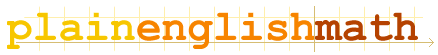You are here: MathematicsFundamentals → Sets

# Number Sets

In mathematics, it is often more useful to talk in terms of sets of numbers rather than just single numbers.

There are two main kinds of set: unordered sets, and ordered sets.

An unordered set is just a list of numbers, for example; {1, 2, 3, 4, 5} is the set of natural counting numbers from 1 to 5. Mathematically, it wouldn't have made any difference if I had written {3, 1, 5, 2, 4} instead, since this is still the same set of numbers, regardless of the order I write them in.

In an ordered set, on the other hand; the order in which each number appears is important, and may even determine what each number in the set means. For example, if a point on a map has coordinates (10, 42), the number 10 might refer to the horizontal co-ordinate on the map, and then the number 42 would be the vertical co-ordinate on the map. If I swap the numbers and write (42, 10) instead; this is obviously a different place on the map.

## Unordered sets

Usually, when a mathematician uses the word "set" by itself, they are talking about an unordered set.
To list an unordered set of numbers, you enclose the set inside curved braces { and }. For example, {12, 0.03, -21.2} is a set of three numbers.
Order doesn't matter, so either a number is inside a set, or it isn't. Each number in a set is called an element. If a number is present in a set, we say that number is an element of that set. The symbol ∈ means "element of", so if we have a set of numbers, and we call that set S; then 15 ∈ S means that 15 is one of the numbers in that set.

## Ordered sets

To list an ordered set of numbers, you use parentheses ( and ). For example, (4, 2) is an ordered set of two numbers (co-ordinates, for instance).
Ordered sets are most often used to describe co-ordinates, such as points on a grid, and more generally; vectors (which are made up of multiple co-ordinates, or "components" corresponding to each axis in space - more on vectors elsewhere on this site).